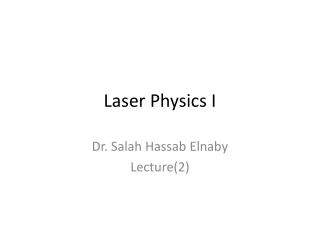Download PresentationLaser Physics I# Laser Physics I - PowerPoint PPT Presentation

Download Presentation##### Laser Physics I

Download Policy: Content on the Website is provided to you AS IS for your information and personal use and may not be sold / licensed / shared on other websites without getting consent from its author. While downloading, if for some reason you are not able to download a presentation, the publisher may have deleted the file from their server.

- - - - - - - - - - - - - - - - - - - - - - - - - - - E N D - - - - - - - - - - - - - - - - - - - - - - - - - - -
##### Presentation Transcript

1. Laser Physics I Dr. SalahHassabElnaby Lecture(2)

2. Energy levels of atoms • Electronic energy • Electric Potential • Orbital motion • Energy levels of molecules • Electronic energy • Vibration energy • Rotational energy

3. Bohr Atomic model Bohr adopted Rutherford’s nuclear model that had been successful in explaining scattering experiments with alpha particles 1910 - 1912. Bohr assumed that almost all the mass of a hydrogen atom is concentrated in a positively charged nucleus, the electron orbits in a cloud. There are two forces Electric force : The centrifugal force F= mv2/r At equilibrium Bohr assumption where L=mvr=nh/2π . so E=T+V =-1/2 mv2

4. For the Hydrogen atom the radius of the atom is Multi electron atoms Pauli Exclusion law No two electrons could have the same quantum state Quantum state – energy -- angular momentum -- spin

5. Quantum States and Degeneracy In the Bohr model a state of the electron is characterized by the quantum number n. However, in the quantum theory a state of the electron is characterized by additional quantum numbers :- (i) Orbital angular momentum there are n possible values of the quantum numberl =0,1, 2, . . . , n-1. (ii) Magnetic quantum number m = – l ,…,-1,0,1,…, l For each l there are 2l +1 possible values of the m. (iii) Spin quantum number , an electron also carries an intrinsic angular momentum, which is called simply spin. S=±1/2h.

6. Therefore, there are 2n2 states associated with each principal quantum number n. Electron state n,l,m,ms Historical designations for the orbital angular momentum quantum numbers are still in use: l= 0 s orbital l= 1 p orbital l= 2 d orbital l= 3 f orbital l= 4 g orbital The first three letters came from the words sharp, principal, and diffuse, which described the character of atomic emission spectra in a qualitative way long before quantum theory showed that they could be associated systematically with different orbital angular momentum values for an electron in the atom.

7. Molecular Energy Levels Elctronic Vibrational Rotational Many vibrational modes Vibrational energy # v Rotational energy # j

8. Vibrational modes of CO2 molecule Asymmetric Stretch ω= 1338 cm-1 Bending mode ω= 667 cm-1 Symmetric Stretch ω= 2349 cm-1

9. CONDUCTORS AND INSULATORS The molecules in liquids and solids are influenced very strongly by their neighbors. What is generally called “solid-state physics” is mostly the study of crystalline solids, that is, solids in which the molecules are arranged in a regular pattern called a crystal lattice. The central fact of the theory of crystalline solids is that the discrete energy levels of the individual atoms are split into energy bands, each containing many closely spaced levels (Fig. 2.11). Between these allowed energy bands are gaps with no allowed energies. The way this happens is easy to explain with a simple example.

10. At room temperature, however, electrons in the valence band may have enough thermal energy to cross the narrow energy gap and go into the conduction band. Thus, diamond, which has a band gap of about 7 eV, is an insulator, whereas silicon, with a band gap of only about 1 eV, is a semiconductor. In a metallic conductor, by contrast, there is no band gap at all; the valence and conduction bands are effectively overlapped.

11. Conduction in metals In a metallic solid the electrons are not all tightly bound at crystal lattice sites. Some of the electrons are free to move over large distances in the metal, much as atoms move freely in a gas. This occurs because metals are formed from atoms in which there are one, two, or occasionally three outer electrons in unfilled configurations. The binding is associated with these weakly held electrons leaving their parent ions and being shared by all the ions, and so we can regard metallic binding as a kind of covalent binding. We can also think of the positive ions as being held in place because their attraction to the “electron gas” exceeds their mutual repulsion.

12. Conduction in Semi-Conductors Semi Conductor becomes conductor by: ===Heating ===Absorption of photons ===Impurities N-type by injection of Phosphorus P-type by injection of Boron

13. P-N Junction

14. Light Emitting Diodes LED An electron can “fall into” a hole, meaning that the electron can replace the missing electron represented by the hole. In doing so the electron becomes part of a covalent bond. This process is called recombination. Now if an electron from the conduction band “recombines” with a hole in the valence band, it loses energy, having been free (E . 0) and then becoming bound (E , 0). Heat : the energy can go to vibrations of the crystal atoms. Radiation : the electron transition is accompanied by the emission of a photon.Thisis analogous to (spontaneous) emission by an atom For silicon, with a gap energy of 1.12 eV, λmax = 1100 nm. For germanium, with Eg = 0.67 eV, l λmax = 1900 nm.